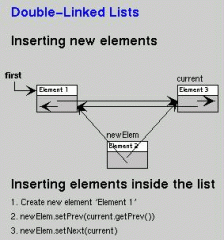•• # Inserting in a Doubly Linked List

## Inserting an Element

To insert an element in the list, the first task is to allocate memory for a new node, assign the element to be inserted to the info field of the node, and then the new node is placed at the appropriate position by adjusting appropriate pointers. Insertion in the list can take place at the following positions:

• At the beginning of the list
• At the end of the list
• After a given element
• Before a given element

## Insertion at the Beginning of the List

First, test whether the linked list is empty, if yes, then the element is inserted as the first and only one element by performing the following steps:

• Assign NULL to the next pointer and prev pointer fields of the new node
• Assign the address of the new node to head and tail pointer variables.

If the list is not empty, then the element is inserted as the first element of the list by performing the following steps:• Assign NULL to the prev pointer field of the new node.
• Assign the value of the head variable (the address of the first element of the existing list) to the next pointer field of the new node.
• Assign the address of the new node to prev pointer field of the node currently pointed by head variable, i.e. first element of the existing list.
• Finally assign the address of the new node to the head variable

## Inserting at the End of the List

First test whether the linked list is initially empty, if yes, then the element is inserted as the first and only one element by performing the following steps:

• Assign NULL value to the next pointer and prev pointer field of the new node
• Assign address of new node to head and tail pointer variable.

If the list is not empty, then element is inserted as the last element of the list by performing the following steps:

• Assign NULL value to the next pointer field of the new node.
• Assign value of the tail variable (the address of the last element of the existing list) to the prev pointer field of the new node.
• Assign address of the new node to the next pointer field of the node currently pointed by tail variable i.e. last element of the existing list.
• Finally assign the address of the new node to tail variable.

## C Code

`void insertatend (node **head, node **tail, int item)`
`{`
`    node *ptr;`
`    ptr = malloc(sizeof(node));`
`    ptr->info = item;`
`    if (*head == NULL)`
`    {`
`        ptr->next = ptr->prev=NULL;`
`        *head = *tail = ptr;`
`    }`
`    else`
`    {`
`        ptr->next=NULL;`
`        ptr->prev=*tail;`
`        (*tail)->next=ptr;`
`        *tail=ptr;`
`    }`
`}`

## Inserting after a Given Element

`void insertafterelement (node **head, node **tail, int item, int after)`
`{`
`    node *ptr, *loc;`
`    ptr = *head;`
`    loc = search(ptr,after);`
`    if(loc == NULL)`
`        return;`
`    ptr=malloc(sizeof(node));`
`    ptr->info = item;`
`    if(loc->next == NULL)`
`    {`
`        ptr->next = NULL;`
`        loc->next = ptr;`
`        ptr->prev = *tail;`
`        *tail = ptr;`
`    }`
`    else`
`    {`
`        ptr->prev = loc;`
`        ptr->next = loc->next;`
`        (loc->next)->prev = ptr;`
`        loc->next = ptr;`
`    }`
`}`

## Inserting before a Given Element

`void insertbeforeelement (node **head, int item, int before)`
`{`
`    node *ptr, *loc;`
`    ptr=*head;`
`    loc=search(ptr,before);`
`    if(loc==NULL)`
`        return;`
`    ptr=malloc(sizeof(node));`
`    ptr->info=item;`
`    if(loc->prev==NULL)`
`    {`
`        ptr->prev=NULL;`
`        loc->prev=ptr;`
`        ptr->next=*head;`
`        *head=ptr;`
`    }`
`    else`
`    {`
`        ptr->prev=loc->prev;`
`        ptr->next=loc;`
`        (loc->prev)->next=ptr;`
`        loc->prev=ptr;`
`    }`
`}`

1. #### Poyraz

23 December, 2010 at 4:37 am

This is AWESOME!! This website is really helpful!